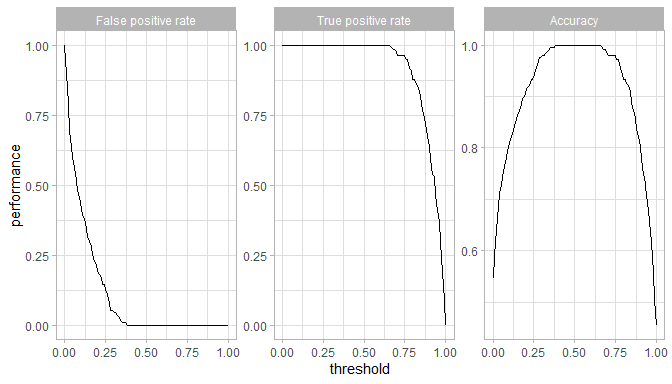# DriveML: Self-Drive machine learning projects

## 1. Introduction

The document introduces the DriveML package and how it can help you to build effortless machine learning binary classification models in a short period.

DriveML is a series of functions such as AutoDataPrep, AutoMAR, autoMLmodel. DriveML automates some of the complicated machine learning functions such as exploratory data analysis, data pre-processing, feature engineering, model training, model validation, model tuning and model selection.

This package automates the following steps on any input dataset for machine learning classification problems

1. Data cleaning
• Replacing NA, infinite values
• Removing duplicates
• Cleaning feature names
2. Feature engineering
• Missing at random features
• Missing variable imputation
• Outlier treatment - Outlier flag and imputation with 5th or 95th percentile value
• Date variable transformation
• Bulk interactions for numerical features
• Frequent transformer for categorical features
• Categorical feature engineering - one hot encoding
• Feature selection using zero variance, correlation and AUC method
3. Binary classification - Model training and validation
• Automated test and validation set creations
• Hyperparameter tuning using random search
• Multiple binary classification algorithms like logistic regression, randomForest, xgboost, glmnet, rpart
• Model validation using AUC value
• Model plots like training and testing ROC curve, threshold plot
• Probability scores and model objects
4. Model Explanation
• Lift plot
• Partial dependence plot
• Feature importance plot
5. Model report
• model output in rmarkdown html format

Additionally, we are providing a function SmartEDA for Exploratory data analysis that generates automated EDA report in HTML format to understand the distributions of the data. Please note there are some dependencies on some other R packages such as MLR, caret, data.table, ggplot2, etc. for some specific task.

To summarize, DriveML package helps in getting the complete Machine learning classification model just by running the function instead of writing lengthy r code.

#### Missing not at random features

Algorithm: Missing at random features

1. Select all the missing features X_i where i=1,2,…..,N.
2. For i=1 to N:
• Define Y_i, which will have value of 1 if X_i has a missing value, 0 if X_i is not having missing value
• Impute all X_(i+1 ) 〖to X〗_(N ) variables using imputation method
• Fit binary classifier f_m to the training data using Y_i ~ X_(i+1 ) 〖+⋯+ X〗_(N )
• Calculate AUC ∝_ivalue between actual Y_i and predicted Y ̂_i
• If ∝_i is low then the missing values in X_i are missing at random,Y_i to be dropped
• Repeat steps 1 to 4 for all the independent variables in the original dataset

## 2. Functionalities of DriveML

The DriveML R package has three unique functions

1. Data Pre-processing and Data Preparation
• autoDataPrep function to generate a novel features based on the functional understanding of the dataset
2. Building Machine Learning Models
• autoMLmodel function to develop baseline machine learning models using regression and tree based classification techniques
3. Generating Model Report
• autoMLReport function to print the machine learning model outcome in HTML format

## 3. Machine learning classfication model use-case using DriveML

This database contains 76 attributes, but all published experiments refer to using a subset of 14 of them. In particular, the Cleveland database is the only one that has been used by ML researchers to this date. The “goal” field refers to the presence of heart disease in the patient. It is integer valued from 0 (no presence) to 4.

Data Source https://archive.ics.uci.edu/ml/datasets/Heart+Disease

Install the package “DriveML” to get the example data set.

library("DriveML")
library("SmartEDA")
## Load sample dataset from ISLR pacakge
data(heart)

more detailed attribute information is there in DriveML help page

### 3.1 Data Exploration

For data exploratory analysis used SmartEDA package

#### Overview of the data

Understanding the dimensions of the dataset, variable names, overall missing summary and data types of each variables

# Overview of the data - Type = 1
ExpData(data=heart,type=1)

# Structure of the data - Type = 2
ExpData(data=heart,type=2)
• Overview of the data
Descriptions Value
Sample size (nrow) 303
No. of variables (ncol) 14
No. of numeric/interger variables 14
No. of factor variables 0
No. of text variables 0
No. of logical variables 0
No. of identifier variables 0
No. of date variables 0
No. of zero variance variables (uniform) 0
%. of variables having complete cases 100% (14)
%. of variables having >0% and <50% missing cases 0% (0)
%. of variables having >=50% and <90% missing cases 0% (0)
%. of variables having >=90% missing cases 0% (0)
• Structure of the data
Index Variable_Name Variable_Type Sample_n Missing_Count Per_of_Missing No_of_distinct_values
1 age integer 303 0 0 41
2 sex integer 303 0 0 2
3 cp integer 303 0 0 4
4 trestbps integer 303 0 0 49
5 chol integer 303 0 0 152
6 fbs integer 303 0 0 2
7 restecg integer 303 0 0 3
8 thalach integer 303 0 0 91
9 exang integer 303 0 0 2
10 oldpeak numeric 303 0 0 40
11 slope integer 303 0 0 3
12 ca integer 303 0 0 5
13 thal integer 303 0 0 4
14 target_var integer 303 0 0 2

#### Summary of numerical variables

ExpNumStat(heart,by="GA",gp="target_var",Qnt=seq(0,1,0.1),MesofShape=2,Outlier=TRUE,round=2)

#### Distributions of Numerical variables

Box plots for all numerical variables vs categorical dependent variable - Bivariate comparison only with classes

Boxplot for all the numerical attributes by each class of the target variable

plot4 <- ExpNumViz(heart,target="target_var",type=1,nlim=3,fname=NULL,Page=c(2,2),sample=8)
plot4[]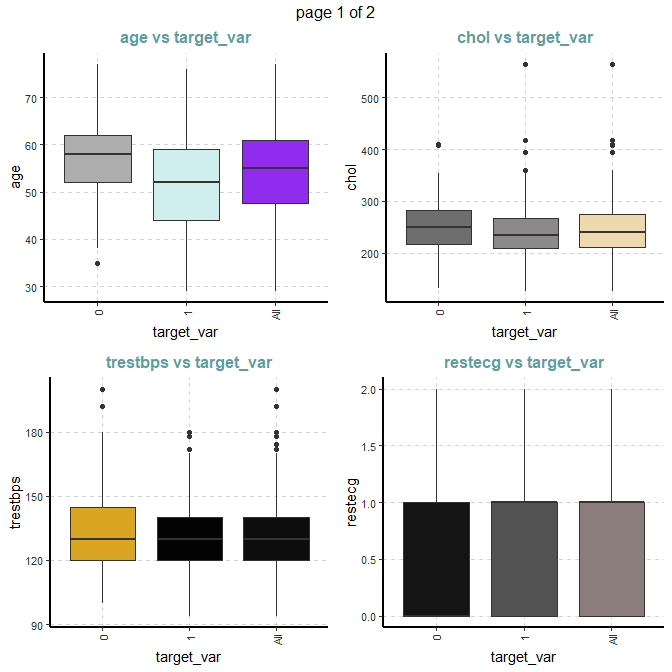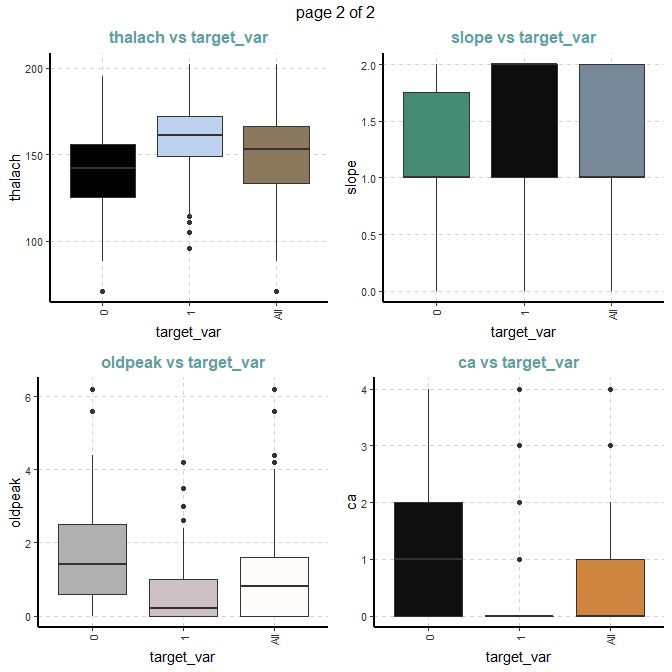#### Summary of categorical variables

Cross tabulation with target_var variable

Custom tables between all categorical independent variables and the target variable

ExpCTable(Carseats,Target="Urban",margin=1,clim=10,nlim=3,round=2,bin=NULL,per=F)
VARIABLE CATEGORY target_var:0 target_var:1 TOTAL
sex 0 24 72 96
sex 1 114 93 207
sex TOTAL 138 165 303
fbs 0 116 142 258
fbs 1 22 23 45
fbs TOTAL 138 165 303
restecg 0 79 68 147
restecg 1 56 96 152
restecg 2 3 1 4
restecg TOTAL 138 165 303
exang 0 62 142 204
exang 1 76 23 99
exang TOTAL 138 165 303
slope 0 12 9 21
slope 1 91 49 140
slope 2 35 107 142
slope TOTAL 138 165 303
target_var 0 138 0 138
target_var 1 0 165 165
target_var TOTAL 138 165 303

#### Distributions of categorical variables

Stacked bar plot with vertical or horizontal bars for all categorical variables

plot5 <- ExpCatViz(heart,target = "target_var", fname = NULL, clim=5,col=c("slateblue4","slateblue1"),margin=2,Page = c(2,1),sample=2)
plot5[]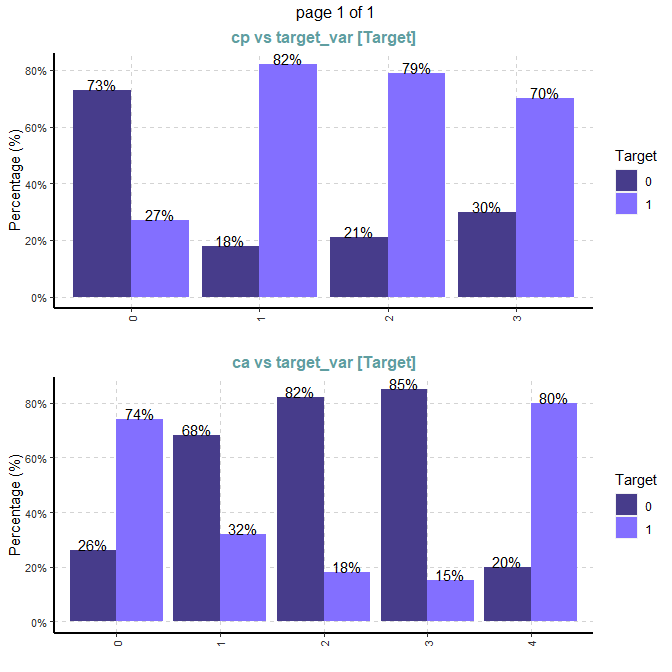#### Outlier analysis using boxplot

ExpOutliers(heart, varlist = c("oldpeak","trestbps","chol"), method = "boxplot",  treatment = "mean", capping = c(0.1, 0.9))
Category oldpeak trestbps chol
Lower cap : 0.1 0 110 188
Upper cap : 0.9 2.8 152 308.8
Lower bound -2.4 90 115.75
Upper bound 4 170 369.75
Num of outliers 5 9 5
Lower outlier case
Upper outlier case 102,205,222,251,292 9,102,111,204,224,242,249,261,267 29,86,97,221,247
Mean before 1.04 131.62 246.26
Mean after 0.97 130.1 243.04
Median before 0.8 130 240
Median after 0.65 130 240

### 3.2 Data preparations using autoDataprep

Data preparation using DriveML autoDataprep function with default options

dateprep <- autoDataprep(data = heart,
target = 'target_var',
missimpute = 'default',
auto_mar = FALSE,
mar_object = NULL,
dummyvar = TRUE,
char_var_limit = 15,
aucv = 0.002,
corr = 0.98,
outlier_flag = TRUE,
uid = NULL,
onlykeep = NULL,
drop = NULL)

train_data <- dateprep$master_data We can use different types of missing imputation using mlr::impute function myimpute <- list(classes=list(factor = imputeMode(), integer = imputeMean(), numeric = imputeMedian(), character = imputeMode())) dateprep <- autoDataprep(data = heart, target = 'target_var', missimpute = myimpute, auto_mar = FALSE, mar_object = NULL, dummyvar = TRUE, char_var_limit = 15, aucv = 0.002, corr = 0.98, outlier_flag = TRUE, uid = NULL, onlykeep = NULL, drop = NULL) train_data <- dateprep$master_data

Adding Missing at Random features using autoMAR function

marobj <- autoMAR (heart, aucv = 0.9, strataname = NULL, stratasize = NULL, mar_method = "glm")
## less than or equal to one missing value coloumn found in the dataframe
dateprep <- autoDataprep(data = heart,
target = 'target_var',
missimpute = myimpute,
auto_mar = TRUE,
mar_object = marobj,
dummyvar = TRUE,
char_var_limit = 15,
aucv = 0.002,
corr = 0.98,
outlier_flag = TRUE,
uid = NULL,
onlykeep = NULL,
drop = NULL)

train_data <- dateprep$master_data ### 3.3 Machine learning models using autoMLmodel Automated training, tuning and validation of machine learning models. This function includes the following binary classification techniques + Logistic regression - logreg + Regularised regression - glmnet + Extreme gradient boosting - xgboost + Random forest - randomForest + Random forest - ranger + Decision tree - rpart mymodel <- autoMLmodel( train = heart, test = NULL, target = 'target_var', testSplit = 0.2, tuneIters = 100, tuneType = "random", models = "all", varImp = 10, liftGroup = 50, maxObs = 4000, uid = NULL, htmlreport = FALSE, seed = 1991) ### 3.3 Model output Model performance Model Fitting time Scoring time Train AUC Test AUC Accuracy Precision Recall F1_score glmnet 2.165 secs 0.007 secs 0.928 0.908 0.820 0.824 0.848 0.836 logreg 2.011 secs 0.004 secs 0.929 0.906 0.820 0.824 0.848 0.836 randomForest 2.257 secs 0.011 secs 1.000 0.874 0.770 0.771 0.818 0.794 ranger 2.312 secs 0.046 secs 1.000 0.896 0.787 0.778 0.848 0.812 xgboost 2.927 secs 0.005 secs 1.000 0.874 0.770 0.757 0.848 0.800 rpart 1.922 secs 0.004 secs 0.927 0.814 0.738 0.730 0.818 0.771 Randomforest model Receiver Operating Characteristic (ROC) and the variable Importance Training dataset ROC TrainROC <- mymodel$trainedModels$randomForest$modelPlots$TrainROC TrainROC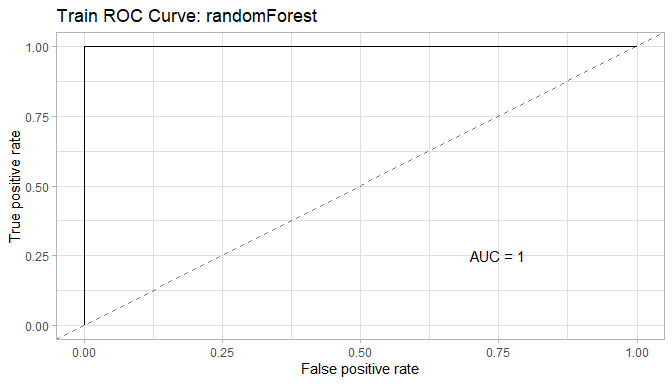Test dataset ROC TestROC <- mymodel$trainedModels$randomForest$modelPlots$TestROC TestROC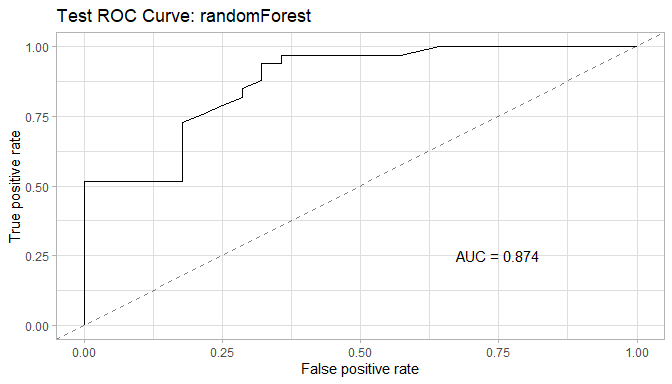Variable importance VarImp <- mymodel$trainedModels$randomForest$modelPlots$VarImp VarImp ## []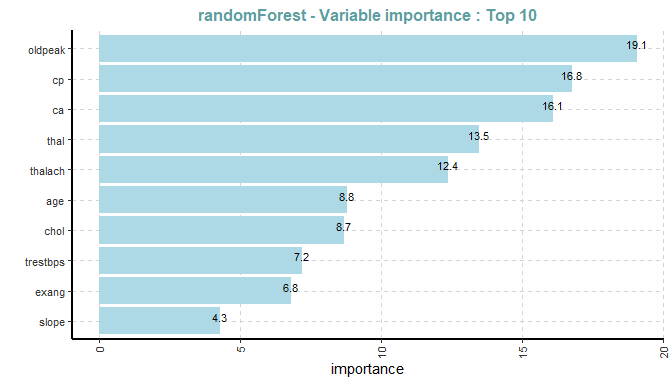Threshold Threshold <- mymodel$trainedModels$randomForest$modelPlots\$Threshold
Threshold